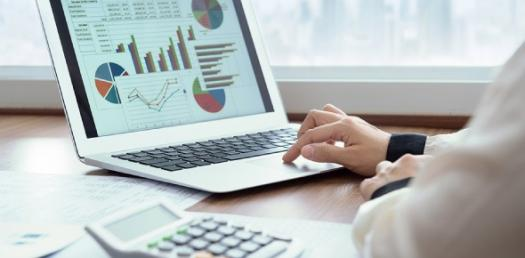# Accounting 201 Chapters 1&2

50 Questions | Attempts: 255SettingsAccounting is one of the complicated subjects to study especially if you are not so good with math. The good news is that, with enough and relevant practice, you will become better. Accounting 201 chapters 1&2 is here for you.

• 1.
Which of the following best describes a liability? Liabilities are:
• A.

A form of paid-in-capital

• B.

Future economic benefits to which a company is entitled

• C.

Debts payable to outsiders called creditors

• D.

Economic obligations to owners to be paid at some future date by the corporation

• 2.
The major types of transactions that affect retained earnings are:
• A.

Paid-in capital and common stock

• B.

Assets and liabilities

• C.

Revenues, expenses, and dividends

• D.

Revenues and liabilities

• 3.
The amount of net income shown on the income statement also appears on the:
• A.

Balance sheet and operations statement

• B.

Statement of assets

• C.

Statement of financial position

• D.

Statement of retained earnings

• 4.
Jason purchased office equipment for \$4,800 on account. This transaction would
• A.

Increase assets and increase owners equity

• B.

Increase assets and increase liabilities

• C.

Increase one asset and decrease another asset

• D.

Decrease assets and decrease liabilities

• 5.
Jason paid \$3,700 on account to the company from which equipment was purchased on credit. This transaction would
• A.

Decrease assets and decrease liabilities

• B.

Increase assets and increase owners equity

• C.

Increase assets and increase liabilities

• D.

Increase one asset and decrease another asset

• 6.
Stephen purchased office supplies for \$800 in cash. This transaction would
• A.

Increase assets and increase owners equity

• B.

Increase one asset and decrease another asset

• C.

Increase assets and increase liabilities

• D.

Decrease assets and decrease liabilities

• 7.
Meghan started her business by investing \$30,000 in cash. This transaction would
• A.

Increase assets and increase owners equity

• B.

Increase assets and increase liabilities

• C.

Increase one asset and decrease another asset

• D.

Decrease assets and decrease liabilities

• 8.
Any accounting period of twelve monts duration is usually referred to as a(n)
• A.

Fiscal year

• B.

Calendar year

• C.

Physical year

• D.

Operating year

• 9.
An example of an expense is
• A.

Investments

• B.

Supplies consumed

• C.

Withdraws by owner

• D.

Prepaid insurance

• 10.
The financial statement that should be comleted first is the
• A.

Balance sheet

• B.

Statement of financial position

• C.

Statement of financial condition

• D.

Income statement

• 11.
Current assets are assets expected to be converted to cash, sold, or consumed within the next:
• A.

12 months

• B.

9 months

• C.

6 months

• D.

24 months

• 12.
The financial statement that shows the state of the firms assets, liablities, and owners equity on a specific date is called a(n)
• A.

Balance sheet

• B.

Statement of operations

• C.

Statement of owners equity

• D.

Income statement

• 13.
A net profit of the business is:
• A.

Expenses are more than revenue

• B.

Revenue is more than expenses

• C.

Revenue is less than expenses

• 14.
Any event that has a financial impact on the business and can be measured is a
• A.

Income statement

• B.

Transaction

• C.

Asset

• D.

Journal

• 15.
Revenues are recorded when
• A.

The company signs a contract

• B.

Work is begun on the job

• C.

Cash is received from the customer

• D.

The work is completed on the job, whether or not the cash is received

• 16.
A company received cash in exchange for issuing stock. This transaction increased assets and
• A.

Increased expenses

• B.

Increased revenues

• C.

Increased liabilities

• D.

Increased equity

• 17.
When a business makes a sale on account, the asset created is a(n)
• A.

Revenue

• B.

Expense

• C.

Account receivable

• D.

Account payable

• 18.
The debit created by a business when it makes a purchase on account is a(n)
• A.

Account receivable

• B.

Revenue

• C.

Prepaid expenses

• D.

Account payable

• 19.
A company paid cash for an amount owed to a creditor. This transaction decreased cash and
• A.

Decreased revenues

• B.

Decreased liabilities

• C.

Decreased expenses

• D.

Increased expenses

• 20.
What type of account is cash?
• A.

A liability

• B.

An expense

• C.

Stock holders equity

• D.

An asset

• 21.
• A.

Increase total assets and decrease total liabilities

• B.

Increase total liabilities and decrease total assets

• C.

Increase total assets and increase total liabilities

• D.

Increase total liabilities and increase stockholders equity

• 22.
A company paid cash for employee wages. This transaction:
• A.

Increased cash and increased expenses

• B.

Increased cash and decreased expenses

• C.

Decreased cash and increased expenses

• D.

Decreased cash and decreased revenues

• 23.
Notes payable, accounts payable, taxes payable and salaries payable are all examples of
• A.

Liabilities

• B.

Revenues

• C.

Expenses

• D.

Assets

• 24.
The left side of a T-acccount is always the:
• A.

Increase side

• B.

Decrease side

• C.

Debit side

• D.

Credit side

• 25.
A listing of all the accounts that make up the ledger is called the:
• A.

T-account

• B.

Ledger

• C.

Journal

• D.

Chart of accounts

## Related TopicsBack to top
×

Wait!
Here's an interesting quiz for you.